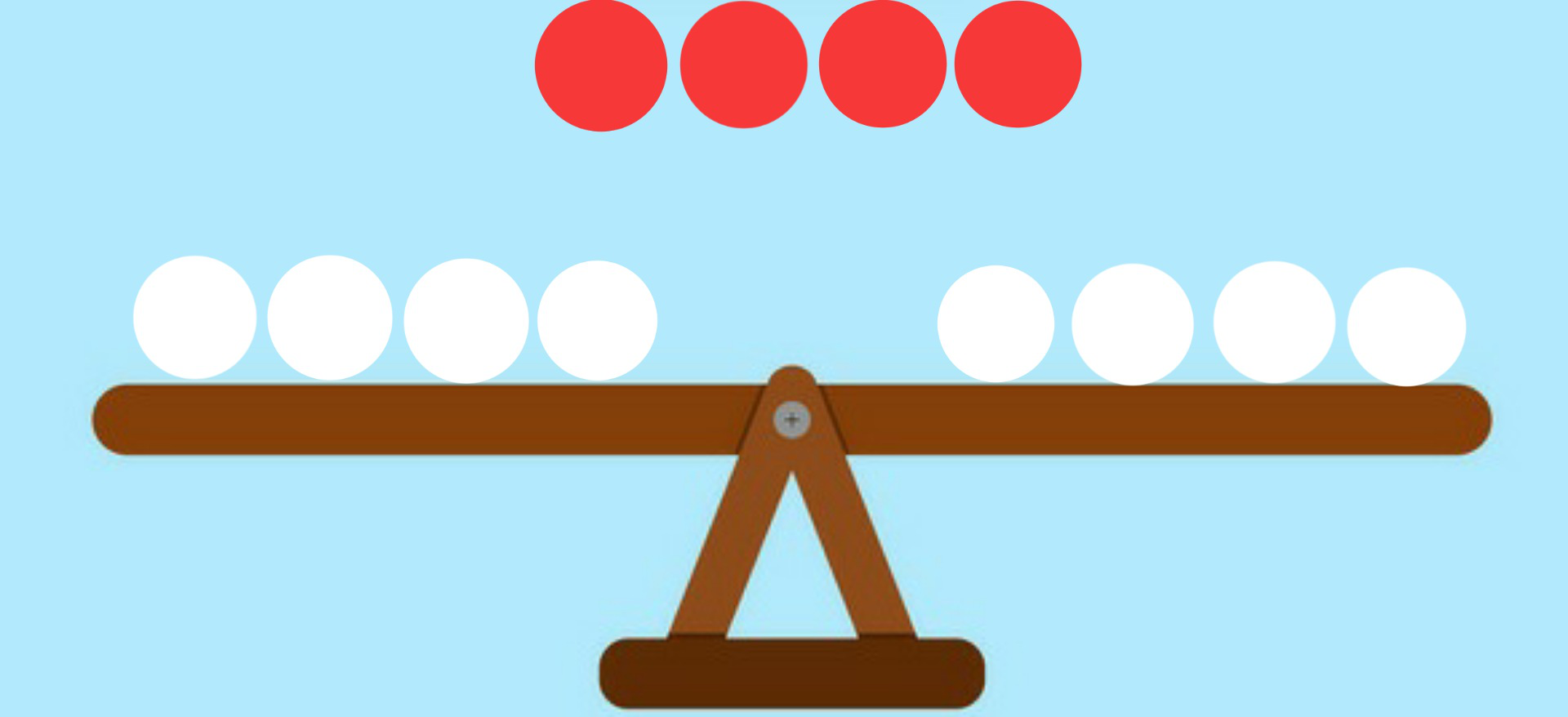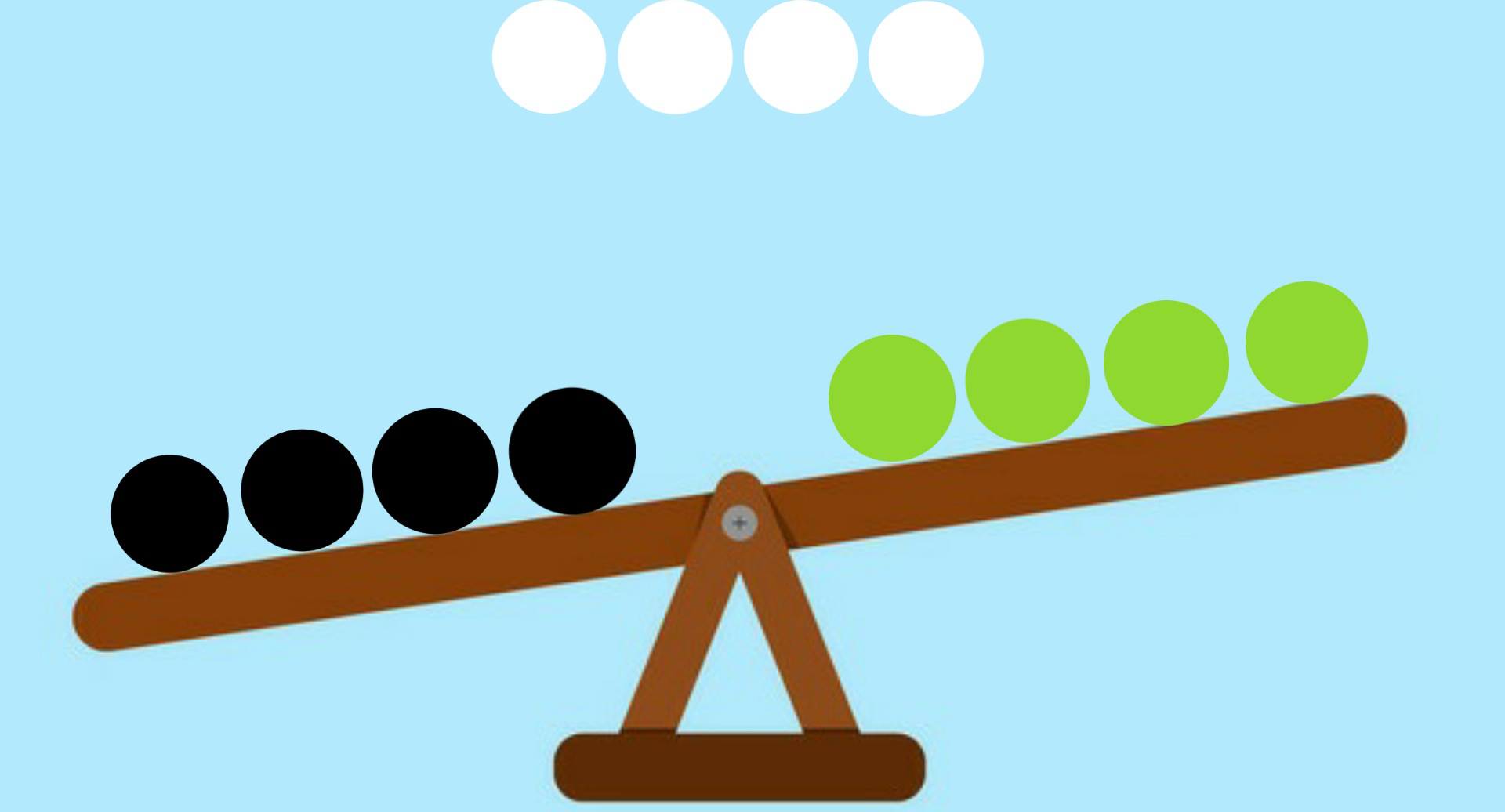GeeksforGeeks App
Open AppBrowser
Continue

# Puzzle | Find the overweight islander

There are 12 men on an island. 11 weigh exactly the same, but one of them is slightly lighter or heavier. There is a seesaw to determine who is the odd one out. Find out the minimum number of times the seesaw will be used.

Solution: The minimum number of times seesaw will be needed is 3.

Explanation: A Divide and Conquer Approach can be used to solve this problem as follows.

Step 1: Divide the 12 men in 3 groups of 4 men each (A, B & C) and weigh the first group (A) against the second group (B).
Step 2: There are 2 possible outcomes.

• Case 1: They balance.
• Case 2: They don’t balance

Case 1This means group C contains the odd one out. Now divide group C in two subgroups of 3 men (C1) and 1 men (C2), weigh the group C1 against 3 normal men from group A or group B and set aside group C2. Again there can be 3 possible outcomes here-

Case 2In this case there are total 8 suspects. Now divide this group in two subgroups of 5 men (A1) and 3 men (B1), Suppose subgroup A1 contains 4 heavier suspects and 1 lighter suspect and subgroup B1 contains 3 lighter suspects. Now compare subgroup A1 in such a way that on one side there are 2 heavier and 1 lighter suspects and on the other side there are the remaining 2 heavier suspects plus one normal men from group C. Again there can be 3 possible outcomes here:

My Personal Notes arrow_drop_up